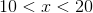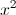## Example Questions

### Example Question #1 : Arithmetic

Neither x nor y is equal to 0.

xy = 4y/x

Quantity A: x

Quantity B: 2

Quantity A is greater.

The relationship cannot be determined from the information given.

The two quantities are equal.

Quantity B is greater.

The relationship cannot be determined from the information given.

Explanation:

Given xy = 4y/x and x and y not 0.

Therefore you are able to divide both sides by 'y' such that:

x = 4/x

Multiply both sides by x:

x2 = 4 or x = +2 or –2.

Because of the fact that x could equal –2, the relationship cannot be determined from the information given.

### Example Question #2 : ArithmeticQuantity A:Quantity B: 399

Quantitiy B is greater.

The relationship cannot be determined from the information given.

Quantity A is greater.

The two quantities are equal.

The relationship cannot be determined from the information given.

Explanation:

Sinceis between 10 and 20, it can be any real number between 100 and 400. Therefore, the relationship cannot be determined sincecould fall anywhere between these two limits, including between 399 and 400.

### Example Question #1 : How To Find The Square Of An Integer

Quantity A: 9

Quantity B: √(25 + 55)

Quantity B is greater.

Quantity A is greater.

The relationship cannot be determined from the information given.

The two quantities are equal.

Quantity A is greater.

Explanation:

In order to determine the relationship between Quantity A and Quantity B, let's convert both to square roots. In order to do this, we must square Quantity A so it becomes √81 which is equivalent to 9. Now to Quantity B, we must simplify by adding the two values together (25 + 55) to get √80.

√81 is greater than the √80 because 81 is greater than 80. Thus Quantity A is greater.

Tired of practice problems?

Try live online GRE prep today.